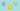Sapnesh Naik
Software Developer, Technical Writer
sapnesh@kerneldev.com

# Format a Number to Indian Rupee Format in PHP or Laravel

November 30, 2017One of the tasks I usually come across while working on some of the more commercial web applications is to display a number in INR i.e Indian National Rupee ( ₹ ) format which makes it really easy to perceive the number as Indian Rupees. Let me show you how to format a number to Indian Rupee format in PHP / Laravel project.

The Indian Rupee Representation uses lakh (1,00,000) and crore (1,00,00,000) so a numerical such as 125520 would be represented as 1,25,520.

This is a function I wrote in `PHP` for use in my `Laravel` project but it can be adapted to work in any programming language you like. The function takes a number as Input and returns the result as a string formatted to INR format.

### The INDmoneyformat() function:

``````<?php
function IND_money_format(\$number){
\$decimal = (string)(\$number - floor(\$number));
\$money = floor(\$number);
\$length = strlen(\$money);
\$delimiter = '';
\$money = strrev(\$money);

for(\$i=0;\$i<\$length;\$i++){
if(( \$i==3 || (\$i>3 && (\$i-1)%2==0) )&& \$i!=\$length){
\$delimiter .=',';
}
\$delimiter .=\$money[\$i];
}

\$result = strrev(\$delimiter);
\$decimal = preg_replace("/0\./i", ".", \$decimal);
\$decimal = substr(\$decimal, 0, 3);

if( \$decimal != '0'){
\$result = \$result.\$decimal;
}

return \$result;
}
?>``````

### What this function actually does:

Here the decimal part(`\$decimal`) of the input number is separately stored as a string which we will use later. We then get rid of the decimal part of the number by using the `floor()` function. This rounds DOWN a number to the nearest integer.

``````\$decimal = (string)(\$number - floor(\$number));
\$money = floor(\$number);``````

We need the `length` of the of the string (`\$money`) which is going to be used in a `for` loop later. We then reverse the original string and also initialize an empty string ( `\$delimiter` ) . This will hold the delimiter ( , ) value as we loop over the reversed string.

``````\$length = strlen(\$money);
\$m = '';
\$money = strrev(\$money);``````

The `for` loop below is the heart of our function. The loop will run as many times as the length of the string and the `if` loop inside `for` loop assigns the delimiter ( , ) to the `\$delimiter` in the cases of:

• `\$i = 3` : The first delimiter will always be placed at the 3rd position (from last i.e end of the string).
• `(( \$i>3 && (\$i-1)%2==0) )&& \$i!=\$length )` : The subsequent delimiters will be placed after every two characters excluding the last character in the string.
• `\$delimiter .=\$money\[\$i\]` : Remember we revered the string in a previous step?. This builds up a new string from that reversed string adding up delimiters in appropriate positions.
``````for(\$i=0;\$i<\$length;\$i++){
if(( \$i==3 || (\$i>3 && (\$i-1)%2==0) )&& \$i!=\$length){
\$delimiter .=',';
}
\$delimiter .=\$money[\$i];
}``````

We then restore the reversed string to its original format and then perform a regular expression search to append a dot ( . ) to the beginning of the decimal string.

We also limit the decimal part to only 3 digits ( can be easily changed according to your requirements).

Then simply concatenate the integer part with the decimal part and return the result!!

``````\$result = strrev(\$delimiter);
\$decimal = preg_replace("/0\./i", ".", \$decimal);
\$decimal = substr(\$decimal, 0, 3);

if( \$decimal != '0'){
\$result = \$result.\$decimal;
}
return \$result;``````

You can also add this function as a global function in Laravel!! see How to add a Global function in Laravel using Composer?

What do you think? Is there a better way of doing this? Please share your thoughts in the comments below!